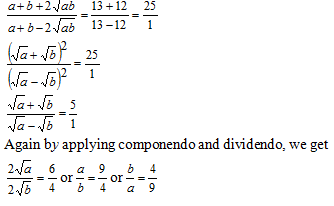Select Page

Introduction:

### Algebra is one of the most important parts of the CAT QA section. Every year, several problems appear from this area. If we look at the overall landscape of the CAT QA section, we can see that the clear majority of the topics are based on topics which we study in class 6 to 10. Algebra is the one differentiator here and a number of topics from class 11/12 appear in the exam.

Question 1: If the harmonic mean between two positive numbers is to their geometric mean as 12 : 13; then the numbers could be in the ratio
(a) 12 : 13
(b) 1/12 : 1/13
(c) 4 : 9
(d) 2 : 3

Option (c)

Let the two positive numbers are ‘a’ and ‘b’. The H.M. between these two numbers is given by (2ab)/(a+b) and the G.M. is given by √ab Now it is given thatApplying componendo and dividendo, we getQuestion 2: 56 – 1 is divisible by
(a) 13
(b) 31
(c) 5
(d) None of these

Option (b)

We have:

56 – 1 = (125)2 – 1 = (125 – 1) (125 + 1) = 124 × 126.

Since 124 is divisible by 31, hence 56 – 1 is divisible by 31.

Question 3: One root of x2 +kx – 8 = 0 is square of the other, then, the value of k is

(a) 2
(b) 8
(c) –8
(d) –2

Option (d)

The given equation is x2 + kx – 8 = 0.

Let its roots be ‘a’ and ‘a2‘.

Now a + a2 = – k ………(i)

and a × a2 = – 8 => a3 = -8 => a = – 2.

Using this value in equation (i), we get,

– 2 + (-2)2 = – k => -k = -2 + 4 => -k = 2 => k = – 2.

Question 4: Once I had been to the post-office to buy stamps of five rupees, two rupees and one rupee. I paid the clerk Rs. 20, and since he did not have change, he gave me three more stamps of one rupee. If the number of stamps of each type that I had ordered initially was more than one, what was the total number of stamps that I bought.

(a) 10
(b) 9
(c) 12
(d) 8

Option (a)

The number of stamps that were initially bought were more than one of each type.

Since the clerk gave me 3 one rupee stamps at the end, it means that I initially bought stamps for Rs17.

Let I initially bought ‘x’ 5 Rs stamps, ‘y’ 2 Rs stamps and ‘z’ one rupee stamps.

So, we have5x + 2y + z = 17.

Now x cannot be three, so x = 2, also if x = 2, then y cannot be 3, otherwise z will be 1.

Hence, y = 2 and z = 3.

So, I bought x + y + z + 3 = 2 + 2 + 3 + 3 = 10 stamps.

Question 5: Given the quadratic equation x2 – (A – 3) x – (A – 7) = 0, for what value of A will the sum of the squares of the roots be zero?

(a) –2
(b) 3
(c) 6
(d) None of these

Option (d)

The given quadratic equation is x2 – (A – 3) x – (A – 7) = 0.

Let the roots be m and n.

So, the sum of the roots is m + n= A – 3

And the product of the roots is mn= – (A – 7)

The sum of the squares of the roots is = m2 + n2 =0

=> (m + n)2 – 2mn = 0

=> (A – 3)2+2(A – 7) = 0

=> A2 + 9 – 6A +2A -14 = 0

=> A2 – 4A -5 = 0

On solving, we get A = 5 or –1.

Tips and Tricks you can use CAT Algebra
• Make sure you clear your basic concepts of algebra first.
• It is important that study the basics of linear and quadratic equations. These are the two most important topics in Algebra and act as the foundation for the topic.
• Functions is an important part of algebra as well. A number of problems appear from this topic in the CAT exam.
• Solving all previous year CAT problems for the topic will help you better grasp the topic and will also help you understand the skills you need to build for this topic.
• You can use class 11/12 books for in-depth understanding of algebra topics. RD Sharma and NCERT books are sufficient for this purpose.

Get Posts Like This Sent to your Email
Updates for Free Live sessions and offers are sent on mail. Don't worry: we do not send too many emails..:)
Get Posts Like This Sent to your Email
Updates for Free Live sessions and offers are sent on mail. Don't worry: we do not send too many emails..:)